Type Package

Title Optimal Stratification of Univariate Populations

Version 1.0-2

Date 2019-09-26

Author Karuna G. Reddy, M.G.M. Khan

Maintainer Karuna G. Reddy <reddy_k@usp.ac.fj>

Description This implements the stratification of univariate populations under stratified sampling designs using the method of Khan et al. (2002) <>, Khan et al. (2008) <> and Khan et al. (2015) <>. It determines the Optimum Strata Boundaries (OSB) and Optimum Sample Sizes (OSS) for the study variable, $$y$$, using the best-fit frequency distribution of a survey variable (if data is available) or a hypothetical distribution (if data is not available). The method formulates the problem of determining the OSB as mathematical programming problem which is solved by using a dynamic programming technique. If a dataset of the population is available to the surveyor, the method estimates its best-fit distribution and determines the OSB and OSS under Neyman allocation directly. When the dataset is not available, stratification is made based on the assumption that the values of the study variable, $$y$$, are available as hypothetical realizations of proxy values of $$y$$ from recent surveys. Thus, it requires certain distributional assumptions about the study variable. At present, it handles stratification for the populations where the study variable follows a continuous distribution, namely, Pareto, Triangular, Right-triangular, Weibull, Gamma, Exponential, Uniform, Normal, Log-normal and Cauchy distributions. In this vignette, the two major functionalities in the package are applied to a number of real and simulated populations and to some hypothetical populations.

LazyData yes

NeedsCompilation yes

Depends R (>= 3.4.4), MASS, fitdistrplus, actuar, triangle, mc2d, zipfR

NeedsCompilation yes

Repository CRAN

Date/Publication 2019-09-26 10:00:00

# 1 Introduction

The main aim of stratification is to produce estimators with a small variance when a population characteristic $$(y)$$ is under study. A simple method that can be used to create strata for this population, if $$y$$ itself is the stratification variable. The ideal situation is that the distribution of such a study variable is known and the OSB can be determined by placing boundaries on the range of this distribution at suitable cut-points. This problem of determining the OSB, when both the estimation and stratification variables are the same, was first discussed by Dalenius (1950). He provided equations for the determination of stratum boundaries that minimize the variance of population estimates under optimal allocation. Dalenius (1957) futher proposed a solution to the problem by taking equal intervals of the cumulative square root of frequency scale of the stratification variable.

One of the many kinds of stratification methods that has been proposed in the literature is due to Bühler and Deutler (1975). They formulated the problem of determining OSB as an optimization problem and developed a computational technique to solve the problem by using Dynamic Programming (DP). A good review of this method can be found in M. G. M. Khan, Nand, and Ahmad (2008). The procedure is also applied by E. A. Khan, Khan, and Ahsan (2002), M. G. M. Khan, Khan, and Ahsan (2003), M. G. M. Khan, Sehar, and Ahsan (2005), M. G. M. Khan, Nand, and Ahmad (2008), M. G. M. Khan, Ahmad, and Khan (2009), M. G. M. Khan et al. (2015), M. G. M. Khan, Reddy, and Rao (2015) for determining OSB for many different distributions. With the known frequency function of the study variable, they considered the problem of finding OSB as an equivalent problem of determining Optimum Strata Width (OSW), which is formulated as a Mathematical Programming Problem (MPP) and solved by using DP technique. They applied the technique to several univariate populations where the study variables followed different probability distributions. In this package, a univariate stratification technique is developed, which is based on the probability distribution assumed by the stratification variable as discussed by the authors above.

As implemented in this package, there are many advantages of using the method. The real advantage of the stratifyR package is that when the dataset of the study variable is not available, which may occur in practice, the package is still able to construct OSB and OSS based on the distributional assumption on the data. Moreover, the population survey can be a costly and time-consuming affair, hence, this approach also has the advantage of determining OSB for the study variable that is not available prior to conducting the survey. This, of course, requires certain distributional or parametric assumptions on the study variables, which can easily be obtained from recent or past surveys.

Other advantages of the method are that it leads to substantial gains in the precision of the estimates over other available methods. Results reveal that the variances get smaller with increasing number of strata $$(L)$$, and they get much smaller at a much faster rate than other available methods. Once the OSB have been determined, the Optimum Sample Sizes (OSS) can be easily calculated for each stratum using Neyman allocation (Neyman (1934)). The algorithm may be a little slow, however, it does provide very efficient results which is probably the most important objective of our survey estimation efforts.

# 2 The Package in a Glance

The stratifyR package implements the DP technique (from various literature by Khan et al) as a stratification procedure for univariate populations, when the stratification variable follows a continuous probability distribution, namely: uniform, triangular, right-triangular, pareto, exponential, normal, log-normal, cauchy, weibull and gamma. The package is able to determine the OSB and OSS from real data and as well as hypothetical situations when the dataset is not available. The latter requires some assumptions about the distribution and its initial value, range, parameter values, fixed sample size, etc.

There are two major functions which basically solve the two types of stratification problems: strata.data() which carries out univariate stratification for those univariate populations where dataset is available and strata.distr() which performs stratification when dataset is not available prior to conducting the survey.

In the former case, data on the study variable, number of strata $$(h)$$, fixed sample size $$(n)$$ and population size $$(N)$$ are used as the input arguments to the strata.data() function in the package. In the latter case, strata.distr() function is called which requires the distribution to be assumed, its parameters, the inital value and the estimated range of the distribution, fixed sample $$(n)$$ and population sizes $$(N)$$. When executed, both the functions output the OSB and OSS, amongst other quantities such as stratum weight $$(W_{h})$$, stratum variance $$(S_{h}^{2})$$, stratum objective function values $$(W_{h}S_{h})$$, stratum sample sizes $$(n_{h})$$, stratum population sizes $$(N_{h})$$ and stratum sampling fraction $$(f_{h})$$.

The following sections show the general formulation of the problem of stratification, the DP solution procedure, the concept of Neyman allocation as the method of determining sample size and the overview of package functionalities. The package is then applied to numerous survey populations with real and simulated data to illustrate its application.

# 3 General Formulation of the Univariate Stratification Problem

Let the target population of the variable under study be stratified into $$L$$ strata where the estimation of the mean of the study variable $$(y)$$ is of interest. If a simple random sample of size $$n_{h}$$ is to be drawn from $$h^{th}$$ stratum with sample mean $$\bar{y}_{h}$$, then the stratified sample mean, $$\bar{y}_{st}$$, is given by $\begin{eqnarray} \bar{y}_{st}=\sum_{h=1}^{L}W_{h}\bar{y}_{h}, \tag{1} \end{eqnarray}$

where $$W_{h}$$ (stratum weight) is the proportion of the population contained in the $$h^{th}$$ stratum.

When the finite population correction factors are ignored, under the Neyman (1934) allocation, the variance of $$\bar{y}_{st}$$ is given by $\begin{eqnarray} Var(\bar{y}_{st})=\dfrac{\left(\sum_{h=1}^{L}W_{h}S_{h}\right)^{2}}{n}, \tag{2} \end{eqnarray}$

where $$S_{h}^{2}$$ is the stratum variance for the study variable in the $$h^{th}$$ (where $$h=1, 2, ..., L$$) stratum and $$n$$ is the preassigned total sample size.

Let $$f(y);\;a\leq y\leq b$$ be the frequency function of the study variable, $$y$$, on which OSB are to be constructed. If the population mean of this study variable is estimated under Neyman allocation, then the problem of determining OSB is to cut up the range, $$d=b-a$$, at $$(L-1)$$ intermediate points $$a=y_{0} \leq y_{1} \leq y_{2} \leq, ..., \leq y_{L-1} \leq y_{L}=b\,$$ such that (2) is minimum. The lower and upper bounds of the study variable are denoted by $$a$$ and $$b$$ respectively and the cut-points $$y_{0}, y_{1}, y_{2}, ..., y_{L-1}$$ are the OSB.

For a fixed sample size $$n$$, minimizing the expression of the right hand side of equation (2) is equivalent to minimizing $\begin{eqnarray} \sum_{h=1}^{L} W_{h}S_{h} \tag{3} \end{eqnarray}$ If $$f(y)$$ is known and integrable, the stratum weight $$(W_{h})$$, stratum variance $$(S_{h}^{2})$$ and stratum mean $$(\mu_{h})$$ can be obtained as a function of the boundary points $$y_{h}$$ and $$y_{h-1}$$ by using the following expressions: $\begin{eqnarray}\label{stratumweight} W_{h} = \int_{y_{h-1}}^{y_{h}} f(y)dy \tag{4} \end{eqnarray}$ $\begin{eqnarray} S_{h}^{2}= \dfrac{1}{W_{h}}\int_{y_{h-1}}^{y_{h}}y^{2}f(y)dy - \mu_{h}^{2} \tag{5} \end{eqnarray}$ $\begin{eqnarray} \textrm{where}\;\;\;\mu_{h}=\dfrac{1}{W_{h}}\int_{y_{h-1}}^{y_{h}}yf(y)dy \tag{6} \end{eqnarray}$

where $$(y_{h-1}, y_{h})$$ are the boundaries of $$h^{th}$$ stratum.

Thus, the objective function in (3) could be expressed as a function of boundary points $$y_{h}$$ and $$y_{h-1}$$ only. We further define $\begin{eqnarray} l_{h}=y_{h}-y_{h-1}; \; h=1,2, ..., L \tag{7} \end{eqnarray}$ where $$l_{h}\geq 0$$ denotes the range or width of the $$h^{th}$$ stratum. Then, the range of the distribution, $$d = b - a$$, is expressed as a function of stratum width as: $\begin{eqnarray}\label{215} \sum_{h=1}^{L}l_{h}=\sum_{h=1}^{L}(y_{h}-y_{h-1}) = b-a = y_{L} - y_{0} = d \tag{8} \end{eqnarray}$ The $$h^{th}$$ stratification point $$y_{h};\;h=1,2,..., L\,$$ is then expressed as $$y_{h}=y_{h-1}+l_{h}$$ and from (8), the problem can be treated as an equivalent problem of determining Optimum Strata Widths (OSW), $$l_{1}, l_{2}, ..., l_{L}$$. Due to the special nature of functions, the problem may be treated as a function of $$l_{h}$$ alone and can be expressed as: $\begin{eqnarray}\label{genMPP} &\textrm{Minimize}& \;\;\; \sum_{h=1}^{L}\phi_{h}(l_{h}),\nonumber \\ &\textrm{subject to}&\;\;\;\; \sum_{h=1}^{L}l_{h} = d,\nonumber \\ &\textrm{and}& \;\;\;\; l_{h} \geq 0; \;\; h = 1,2,...,L. \tag{9} \end{eqnarray}$

# 4 Dynamic Programming Technique as a Solution Procedure

Many multistage decision problems can be formulated as a Mathematical Programming Problem (MPP). The Dynamic Programming technique, developed by Bellman, R E (1957), is a computational method which is well suited for solving MPPs that may be treated as a multistage decision problem. The DP technique determines the optimum solution of a multistage problem by decomposing it into stages, each stage comprising of a single stage. The advantage of the decomposition is that the optimization process at each stage involves one variable only, which simplifies the computational task by dealing with all variables simultaneously.

The solution to an MPP is achieved in a sequential manner starting from one stage problem, moving onto a two stage problem, to a three stage problem and so on until finally all stages are included. The solution for $$n$$ stages is obtained by adding the $$n^{th}$$ stage to the solution of $$n - 1$$ stages.

The basic concept of DP technique is contained in the principle of optimality proclaimed by Bellman, R E (1957), which implies that given the initial state of a system, an optimal policy for the subsequent stages does not depend upon the policy adopted at the preceding stages. It determines the optimum solution of a multi-variable problem by decomposing it into stages, each stage compromising a single variable sub-problem. A dynamic programming model is basically a recursive equation which links the different stages of the problem in a manner which guarantees that each stage’s optimal feasible solution is also optimum and feasible for the entire problem.

Consider the following sub-problem of (9) for first $$k(<L)$$ strata:

$\begin{eqnarray} &\text{Minimize}& \;\;\;\; \sum_{h=1}^{k}\phi_{h}(l_{h}),\nonumber \\ &\text{subject to}&\;\;\;\;\; \sum_{h=1}^{k}l_{h} = d_{k},\nonumber \\ &\text{and}& \;\;\;\;\; l_{h} \geq 0; \;\; h = 1,2,...,k.\tag{10} \end{eqnarray}$

where $$d_{k}< d$$ is the total width available for division into strata or the state value at stage $$k$$. Note that $$d_{k} = d$$ for $$k = L$$.

The transformation functions are given by:

$\begin{eqnarray*} d_{k}\;\;&=&\;\;l_{1}+l_{2}+...+l_{k},\\ d_{k-1}\;\;&=&\;\;l_{1}+l_{2}+...+l_{k-1}\;=\;d_{k}-l_{k},\\ d_{k-2}\;\;&=&\;\;l_{1}+l_{2}+...+l_{k-2}\;=\;d_{k-1}-l_{k-1},\\ &\vdots&\;\;\;\;\;\;\;\;\;\;\;\;\vdots\\ d_{2}\;\;&=&\;\;l_{1}+l_{2}\;=\;d_{3}-l_{3},\\ d_{1}\;\;&=&\;\;l_{1}\;=\;d_{2}-l_{2}. \end{eqnarray*}$

Let $$\Phi_{k}(d_{k})$$ denote the minimum value of the objective function of MPP (10), that is,

$\begin{equation} \Phi_{k}(d_{k}) = \text{min}\left[ \sum_{h=1}^{k}\phi_{h}(l_{h})\bigg|\sum_{h=1}^{k}l_{h}=d_{k}, \,\textrm{and}\,l_{h}\;\geq 0;\,h=1,2,...,k \;\textrm{and}\; 1\leq k \leq L \right].\nonumber \end{equation}$

With the above definition of $$\Phi_{k}(d_{k})$$, (10) is equivalent to finding $$\Phi_{L}(d)$$ recursively by finding $$\Phi_{k}(d_{k})$$ for $$k = 1, 2, ..., L$$ and $$0 \leq d_{k} \leq d.$$

We can write:

$\begin{equation} \Phi_{k}(d_{k}) = \text{min}\left[\phi_{k}(l_{k})+ \sum_{h=1}^{k-1}\phi_{h}(l_{h})\bigg|\sum_{h=1}^{k-1}l_{h}=d_{k}-l_{k}, \;\textrm{and}\;l_{h}\;\geq\;0;\;h=1,2,...,k\right].\nonumber \end{equation}$

For a fixed value of $$l_{k}$$; $$0 \leq l_{k} \leq d_{k}$$,

$\begin{equation} \Phi_{k}(d_{k}) = \phi_{k}(l_{k})+ \text{min}\left[ \sum_{h=1}^{k-1}\phi_{h}(l_{h})\bigg|\sum_{h=1}^{k-1}l_{h}=d_{k}-l_{k}, \;\textrm{and}\;l_{h}\;\geq 0;\;h=1,2,...k-1\;\textrm{and}\;\; 1\leq k \leq L\right].\nonumber \end{equation}$

Using the Bellman’s principle of optimality, a forward recursive equation can be written as:

$\begin{equation} \Phi_{k}(d_{k}) = {\text{min} \atop 0 \leq l_{k} \leq d_{k}}\left[\phi_{k}(l_{k}) + \Phi_{k-1}(d_{k}-l_{k})\right],\;\;k\;\geq\;2.\tag{11} \end{equation}$

For the first stage, that is, for $$k=1$$:

$\begin{equation} \Phi_{1}(d_{1}) = \phi_{1}(d_{1})\; \Longrightarrow\;\;l_{1}^{*}=d_{1},\tag{12} \end{equation}$

where $$l_{1}^{*}=d_{1}$$ is the optimum width of the first stratum. The relations (11) and (12) are solved recursively for each $$k=1, 2, ..., L\,$$ and $$0 \leq d_{k} \leq d$$, and $$\Phi_{L}(d)$$ is obtained. From $$\Phi_{L}(d)$$ the optimum width of $$L^{th}$$ stratum, $$l_{L}^{*}$$, is obtained. From $$\Phi_{L-1}(d - l_{L}^{*})$$ the optimum width of $$(L - 1)^{th}$$ stratum, $$l_{L-1}^{*}$$, is obtained and so on until $$l_{1}^{*}$$ is obtained.

The above algorithm of the Dynamic Programming solution procedure to solve the MPP given in (9) is summarized with the following steps:

1. Start at $$k=1$$. Set $$\Phi_{0}(d_{0})=0$$.
2. Calculate $$\Phi_{1}(d_{1})$$, the minimum value of RHS of $$(12)$$ for $$l_{1}=d_{1},\; 0\leq l_{1}\leq d_{1}$$, and $$0\leq d_{1}\leq d$$.
3. Record $$\Phi_{1}(d_{1})$$ and $$l_{1}$$.
4. For $$k=2$$, express the state variable as $$d_{k-1}=d_{k}-l_{k}$$.
5. Set $$\Phi_{k}(d_{k})=0$$ if $$l_{k}>d_{k}$$, where $$0\leq d_{k}\leq d$$.
6. Calculate $$\Phi_{k}(d_{k})$$, the minimum value of RHS of $$(11)$$ for $$l_{k};\;0\leq l_{k}\leq d_{k}$$.
7. Record $$\Phi_{k}(d_{k})$$ and $$l_{k}$$.
8. For $$k\geq3, ..., L$$, go to Step 4.
9. At $$k=L, \; \Phi_{L}(d)$$ is obtained and hence the optimum value $$l_{L}^{*}$$ of $$l_{L}$$ is obtained.
10. At $$k=L-1$$, using the backward calculation for $$d_{L-1}=d-l_{L}^{*}$$, read the value of $$\Phi_{L-1}(d_{L-1})$$ and hence the optimum value $$l_{L-1}^{*}$$ of $$l_{L-1}$$.
11. Repeat Step $$(10)$$ until the optimum value $$l_{1}^{*}$$ of $$l_{1}$$ is obtained from $$\Phi_{1}(d_{1})$$.

# 5 Optimum Sample Sizes Using Neyman Allocation

When OSB $$(y_{h}, y_{h-1})$$ have been determined, the Optimum Sample Sizes (OSS) $$n_{h}; h=1,2,...,L\,$$ that minimizes the variance of the estimate can easily be computed. The sample size $$n_{h}$$ are obtained for a fixed total sample of size $$n$$ under the Neyman allocation for $$h=1,2,...,L\,$$ and given as follows:

$\begin{equation}\label{Ney_alloc} n_{h} = n\,\frac{W_{h}S_{h}}{\sum_{h=1}^{L}W_{h}S_{h}} \tag{13} \end{equation}$

where $$W_{h}$$ and $$S_{h}$$ are derived in terms of the optimum boundary points $$(y_{h}, y_{h-1})$$.

In Neyman allocation, the total sample size is proportional to the stratum size multiplied by the standard deviation of the stratum. If the variances are specified correctly, Neyman allocation will give an estimator with smaller variance compared to proportional allocation (Lohr (2009)).

In equation (13), it is also worth noting that the OSB $$(y_{h}, y_{h-1})$$ are so obtained that $$n_{h}$$ must satisfy the restrictions:

$\begin{equation}\label{res} 1\leq n_{h}\leq N_{h}, \tag{14} \end{equation}$

where $$N_{h}=NW_{h}$$. Thus, the restriction (14) indicates that the $$h^ {th}$$ stratum must form with at least one unit and also avoid the problem of over-sampling.

# 6 Overview of Package Functionalities

For the numerical illustrations and application of the package, some of the real datasets such as ‘sugarcane’ of M. G. M. Khan, Reddy, and Rao (2015), ‘anaemia’ of Reddy, Khan, and Rao (2014); ‘hies’ and ‘math’ data are provided in the stratifyR. The ‘quakes’ and ‘Boston’ data provided in the datasets package in R are also used for illustration purposes. The stratifyR package is also tested on some published and commonly-used datasets such as ‘UScities’ and ‘UScolleges’ data from Cochran (1961), ‘Debtors’ data of Gunning and Horgan (2004), ‘REV84’ variable for ‘Swedish municipalities’ data from Särdnal, Swensson, and Wretman (1992) and ‘MRTS’ variable from ‘Statistics Canada Monthly Retail Trade Survey’ together with ‘SHS’ data collected in ‘Statistics Canada Survey of Household Spending’. For those distributions where real data is not found in literature, data is simulated to demonstrate the application of the package in this documentaion.

For a user, there are two different routes available in the package and these are basically dependent on the type of stratification problem to be solved. It could either be a data-based (i.e., when the dataset of the stratification variable is available) or a distribution-based (i.e., when dataset is not available) stratification problem. Whether stratification is based on data or not, the idea is that the problem is formulated as an MPP using the estimated (with available data) or assumed (with unavailable data) distribution of the data set. There are numerous functions created in the package, however, there are only a few major functions that are used by the two different types of problems being studied in univariate stratification.

If it is a data-based problem, the function used is strata.data() and the user has to provide as input arguments: the data, the number of strata ($$L$$) and the fixed sample size ($$n$$). For the distribution-based problem, the function used is strata.distr() and the user has to provide the name of the assumed distribution, number of strata ($$L$$), possible range of data (distance), fixed sample size ($$n$$) and the population size ($$N$$). The following two subsections delve a little deeper into the workings surrounding the two functions: strata.data() and strata.distr().

## 6.1 The Function strata.data()

This function computes the OSB, OSS, and other important quantities from univariate survey populations by employing the methodology proposed by E. A. Khan, Khan, and Ahsan (2002) M. G. M. Khan, Khan, and Ahsan (2003), M. G. M. Khan, Sehar, and Ahsan (2005), M. G. M. Khan, Nand, and Ahmad (2008), M. G. M. Khan, Ahmad, and Khan (2009), Nand and Khan (2009), M. G. M. Khan et al. (2015), M. G. M. Khan, Reddy, and Rao (2015), Karuna Garan Reddy, Khan, and Khan (2018) and Karuna G Reddy and Khan (2019). Their method uses the estimated distribution of the data and formulates the problem of determining OSB as a Mathematical Programming Problem which is an optimization problem that is solved by the DP technque. The OSB obtained are optimal solutions that are used to calculate the OSS under Neyman allocation. The usage and arguments are as follows:

    strata.data(data, h, n, cost=FALSE, ch=NULL)

    data - A vector: data containing every unit of the survey population
h - A numeric: number of strata to be sampled. The default is 2
n - A numeric: fixed total sample size
cost - A logical: stratum cost. Default cost=FALSE.
ch - A numeric: denotes a vector of stratum costs. Default ch=NULL.


To show the application of the strata.data() function, an example of the command used and its output from the package is given below. The problem uses the ‘mag’ variable from the ‘quakes’ data (with a population of $$N=1000$$) available from the datasets package in R. To construct a 2-strata solution with a fixed sample size of $$n=300$$, we use the following codes:

data(quakes)
#>      lat   long depth mag stations
#> 1 -20.42 181.62   562 4.8       41
#> 2 -20.62 181.03   650 4.2       15
#> 3 -26.00 184.10    42 5.4       43
#> 4 -17.97 181.66   626 4.1       19
#> 5 -20.42 181.96   649 4.0       11
#> 6 -19.68 184.31   195 4.0       12
mag <- quakes$mag length(mag) #>  1000 hist(mag) #to see the distribution library(stratifyR) #> Loading required package: fitdistrplus #> Loading required package: MASS #> Loading required package: survival #> Loading required package: npsurv #> Loading required package: lsei #> Loading required package: zipfR #> Loading required package: actuar #> #> Attaching package: 'actuar' #> The following object is masked from 'package:grDevices': #> #> cm #> Loading required package: triangle #> Loading required package: mc2d #> Loading required package: mvtnorm #> #> Attaching package: 'mc2d' #> The following objects are masked from 'package:base': #> #> pmax, pmin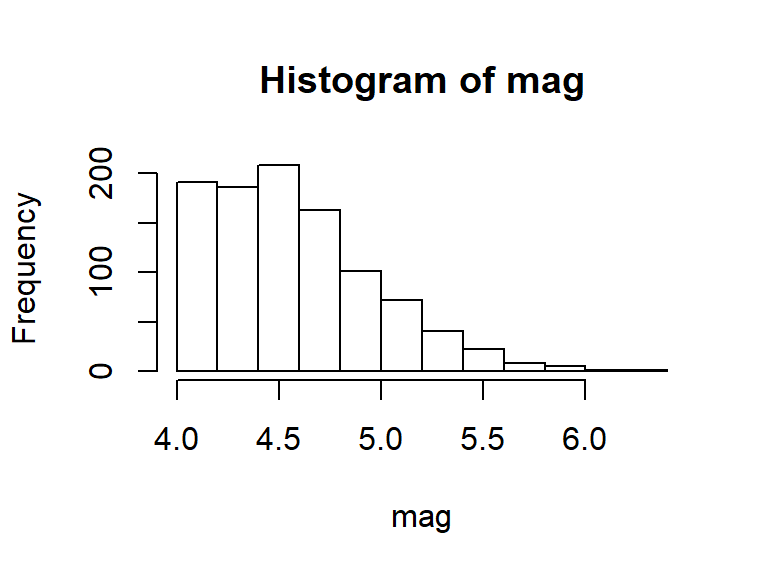res <- strata.data(mag, h = 2, n=300) # a 2-strata solution #> The program is running, it'll take some time! summary(res) #> _____________________________________________ #> Optimum Strata Boundaries for h = 2 #> Data Range: [4, 6.4] with d = 2.4 #> Best-fit Frequency Distribution: lnorm #> Parameter estimate(s): #> meanlog sdlog #> 1.52681032 0.08503554 #> ____________________________________________________ #> Strata OSB Wh Vh WhSh nh Nh fh #> 1 4.68 0.58 0.03 0.109 140 585 0.24 #> 2 6.4 0.42 0.09 0.124 160 415 0.38 #> Total 1.00 0.12 0.233 300 1000 0.30 #> ____________________________________________________ All calculations have been rounded off to 2 decimal places, hence, the individual stratum solutions provided in the tables may not always add up to the totals. ## 6.2 The Function strata.distr() This function is also used to compute the OSB, OSS, and other important quantities from univariate survey populations by employing the methodology proposed by Khan et al given earlier. Its algorithm is quite similar to that of the strata.data(), however, its functionality is applied to the case where the dataset of the population is not available and the distributonal assumptions of the study variable are strictly required. Another caveat for such distribution-based stratification is that the distr.alloc() function uses the probability density functions of the assumed distributions and integration rules presented by equations (4)-(6) to calculate the stratum sample sizes. It must be noted that this function works on ideal distributions that assumes the parameters chosen by the user. The usage and arguments are as follows:  strata.distr(h, initval = NULL, dist = NULL, distr = c("pareto", "triangle", "rtriangle", "weibull", "gamma", "exp", "unif","norm", "lnorm", "cauchy"), params = c(shape=0, scale=0, rate=0, gamma=0, location=0, mean=0, sd=0, meanlog=0, sdlog=0, min=0, max=0, mode=0), n, N, cost=FALSE, ch=NULL)  h - A numeric: number of strata to be sampled initval - A numeric: initial value of the assumed distribution dist - A numeric: distance or range of the assumed distribution distr - A character: the assumed distribution of the hypothetical population params - A list: parameters of the assumed distribution n - A numeric: fixed total sample size N - A numeric: fixed population size cost - A logical: stratum cost. Default cost=FALSE. ch - A numeric: denotes a vector of stratum costs. Default ch=NULL.  The algorithm for strata.distr() is quite similar to the strata.data() for the contruction of OSB. It is only at the sample allocation (OSS) stage that the two are different. strata.distr() is the main function and once the OSB have been computed, this function uses the distr.alloc() function which uses the numerical integration rules for different distibutions (i.e., the equations (4)-(6)) to compute the OSS. To show the application of the strata.distr() function, let us construct a 2-strata solution assuming that the dataset of the stratification variable is not available but its distribution and estimated parameters are. Let us consider the ‘depth’ variable from the ‘quakes’ dataset from the datasets package, which has a Triangular distribution with parameters $$min=39.99998, max=680, mode=39.99999$$. It starts at an initial value of $$40$$ and has a distance (range) of $$640$$ with a fixed sample size of $$n=300$$ from a population of $$N=1000$$ seismic events. Thus, we use the following commands: data(quakes) depth <- quakes$depth
hist(depth) #see distribution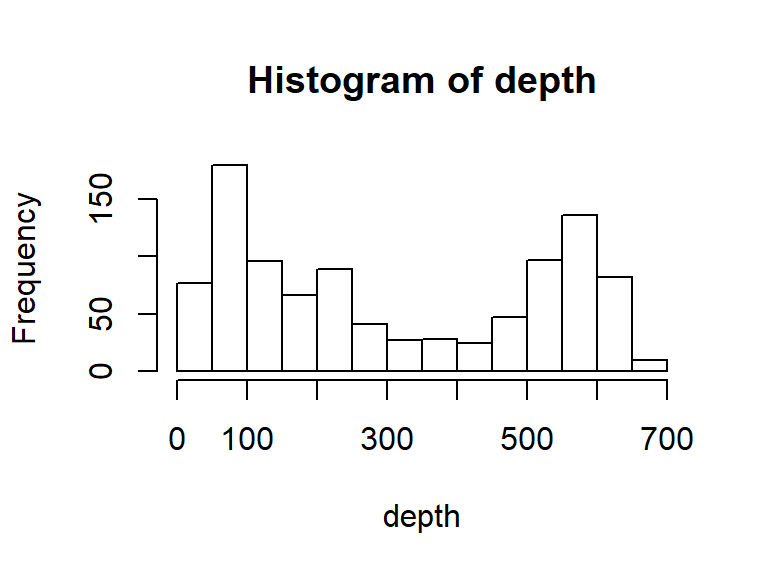min(depth); max(depth); d=max(depth)-min(depth);d #min, max and range of data
#>  40
#>  680
#>  640
# the 2-strata solution is
res <- strata.distr(h=2, initval=40, dist=640, distr = "triangle",
params = c(min=39.99998, max=680, mode=39.99999), n=300, N=1000)
#> The program is running, it'll take some time!
summary(res)
#> _____________________________________________
#> Optimum Strata Boundaries for h = 2
#> Data Range: [40, 680] with d = 640
#> Best-fit Frequency Distribution:  triangle
#> Parameter estimate(s):
#>       min       max      mode
#>  39.99998 680.00000  39.99999
#> ____________________________________________________
#>  Strata    OSB   Wh       Vh   WhSh  nh   Nh   fh
#>       1 266.72 0.58  4217.46 37.862 145  583 0.25
#>       2    680 0.42  9488.76 40.619 155  417 0.37
#>   Total        1.00 13706.22 78.481 300 1000 0.30
#> ____________________________________________________

# 7 Application to Numerous Survey Populations

As discussed earlier, the stratifyR package is able to handle ten continuous distributions that are quite commonly-used in real-life situations. This section presents a brief overview of these distributions and the application of the proposed method of stratification using real or simulated data which follows a particular distribution. Examples for hypothetical distributions are also presented. For the sake of brevity, the mathematical formulations of the problem of determining the OSB and the DP solution procedure are presented only for the Pareto Type II variable. For all other distributions, only the examples are presented to illustrate the application of the package.

## 7.1 Stratification for a Survey Variable with Pareto Type II Distribution

Let the study variable follow the Pareto Type II distribution on the domain of [$$0, +\infty$$], its two-parameter probability density function with a state space $$y\geq 0$$ is given by: $\begin{equation} f(y; s,a)=\dfrac{a\,s^{a}}{(y+s)^{a+1}}, \;\;\;\;\;a,s > 0 \tag{15} \end{equation}$

where $$\alpha > 0$$ is the shape parameter and $$s>0$$ is the scale parameter of the distribution.

### 7.1.1 MPP Formulation for Pareto Type II Distribution

If the study variable $$y$$ follows Pareto II (or Lomax) distribution (i.e., $$y\sim P(a, s)$$) with density function given by (15). By using equations (4)-(6), the formulated MPP given in (10) could be expressed as: $\begin{eqnarray} \textrm{Minimize} \;\;\; \sum_{h=1}^{L} \textit{SQRT} && \Biggl\{as^{2a} \left[\dfrac{(y_{h-1}+l_{h}+s)^{a}-(y_{h-1}+s)^{a}}{(y_{h-1}+s)^{a}(y_{h-1}+l_{h}+s)^{a}}\right] \nonumber \\[10pt] && \times\left[\dfrac{(y_{h-1}+l_{h}+s)^{2-a}}{2-a} - \dfrac{2s(y_{h-1}+l_{h}+s)^{1-a}}{1-a} \right. \nonumber\\[10pt] && - \dfrac{s^2(y_{h-1}+l_{h}+s)^{-a}}{a} -\dfrac{(y_{h-1}+s)^{2-a}}{2-a}\nonumber\\[10pt] && + \left. \dfrac{2s(y_{h-1}+s)^{1-a}}{1-a} + \dfrac{s^2(y_{h-1}+s)^{-a}}{a}\right] \nonumber\\[10pt] && \times \dfrac{s^{2a}}{(1-a)^{2}}\left[\dfrac{a(y_{h-1}+l_{h})+s}{(y_{h-1}+l_{h}+s)^{a}} - \dfrac{ay_{h-1}+s}{(y_{h-1}+s)^{a}} \right]^{2}\Biggr\}\nonumber\\[15pt] \textrm{subject to} && \;\;\;\; \sum_{h=1}^{L}l_{h} = d,\nonumber \\ \textrm{and} && \;\;\;\; l_{h} \geq 0; \;\; h = 1,2,...,L. \tag{16} \end{eqnarray}$

where $$d=y_{L}-y_{0}$$, $$a$$ and $$s$$ are parameters of the Pareto Type II distribution.

### 7.1.2 DP Solution for Pareto Type II Distribution

To solve the MPP (16) using the DP technique as a solution procedure, we apply the algorithm, that is, the solution proceure using Dynamic Progrmming technique discussed earlier in Section 4. After substituting the quatity $$y_{h-1}=y_{0} + d_{h} - l_{h}$$, the recurrence relations (11) and (12) are reduced to:

For the first stage, $$k=1$$, at $$l_{1}^{*}=d_{1}$$:

$\begin{eqnarray} \Phi_{1}d_{1}&=& \textrm{Sqrt}\Biggl\{ as^{2a}\left[\dfrac{(d_{1}+y_{0}+s)^{a}-(y_{0}+s)^{a}}{(y_{0}+s)^{a}(d_{1}+y_{0}+s)^{a}}\right] \nonumber\\[10pt] &&\times\left[\dfrac{(d_{1}+y_{0}+s)^{2-a}}{2-a} - \dfrac{2s(d_{1}+y_{0}+s)^{1-a}}{1-a} \right. \nonumber\\[10pt] &&- \dfrac{s^2(d_{1}+y_{0}+s)^{-a}}{a} -\dfrac{(y_{0}+s)^{2-a}}{2-a}\nonumber\\[10pt] &&+\left. \dfrac{2s(y_{0}+s)^{1-a}}{1-a} + \dfrac{s^2(y_{0}+s)^{-a}}{a}\right] \nonumber\\[10pt] &&\times \dfrac{s^{2a}}{(1-a)^{2}}\left[\dfrac{a(d_{1}+y_{0})+s}{(d_{1}+y_{0}+s)^{a}} - \dfrac{ay_{0}+s}{(y_{0}+s)^{a}} \right]^{2}\Biggr\} \tag{17} \end{eqnarray}$

And for the stages $$k\geq2$$:

$\begin{eqnarray} \Phi_{k}d_{k}&=&\min_{0\leq l_{k}\leq d_{k}}\Biggl\{ \textrm{Sqrt}\Biggl\{ as^{2a}\left[\dfrac{(d_{k}+y_{0}+s)^{a}-(d_{k}+l_{k}+y_{0}+s)^{a}}{(d_{k}+l_{k}+y_{0}+s)^{a}(d_{k}+y_{0}+s)^{a}}\right] \nonumber\\[10pt] &&\times\left[\dfrac{(d_{k}+y_{0}+s)^{2-a}}{2-a} - \dfrac{2s(d_{k}+y_{0}+s)^{1-a}}{1-a} \right. \nonumber\\[10pt] &&- \dfrac{s^2(d_{k}+y_{0}+s)^{-a}}{a} -\dfrac{(d_{k}+l_{k}+y_{0}+s)^{2-a}}{2-a}\nonumber\\[10pt] &&+\left. \dfrac{2s(d_{k}+l_{k}+y_{0}+s)^{1-a}}{1-a} + \dfrac{s^2(d_{k}+l_{k}+y_{0}+s)^{-a}}{a}\right] \nonumber\\[10pt] &&\times \dfrac{s^{2a}}{(1-a)^{2}}\left[\dfrac{a(d_{k}+y_{0})+s}{(d_{k}+y_{0}+s)^{a}}- \dfrac{a(d_{k}+l_{k}+y_{0})+s}{(d_{k}+l_{k}+y_{0}+s)^{a}} \right]^{2} \Biggr\} + \Phi_{k-1}(d_{k}-l_{k})\Biggr\}. \tag{18} \end{eqnarray}$

The recurrence relations (17) and (18) are solved using the DP technique to determine the OSB.

### 7.1.3 A Numerical Example for Pareto Type II Distribution

A dataset for a univariate population of size $$N=5000$$ with the study variable that follows Pareto Type II distribution ($$pareto\_data$$) was simulated using parameters $$shape=5$$ and $$scale=8$$ to demonstrate the application of the strata.data() function to determine the OSB and other quantites. The data exhibits a 2-parameter Pareto Type II distribution with the MLE estimates of the parameters as $$shape=5.026907$$ and $$scale=8.191676$$. The minimum and maximum values in the simulated data are $$[y_{0}, y_{L}] = [0.0002193, 38.56871]$$, which implies that $$d=38.56849$$.

To construct the OSB (a 2-strata solution, i.e., $$h = 2$$) for the $$pareto\_data$$ with a fixed total sample size of $$500$$, we use the following codes:

set.seed(8235411)
pareto_data <- rpareto(5000, shape=5, scale=8)
#>  1.8464786 0.9226109 1.1079785 9.7699864 4.5600912 0.0441124
hist(pareto_data, breaks=100)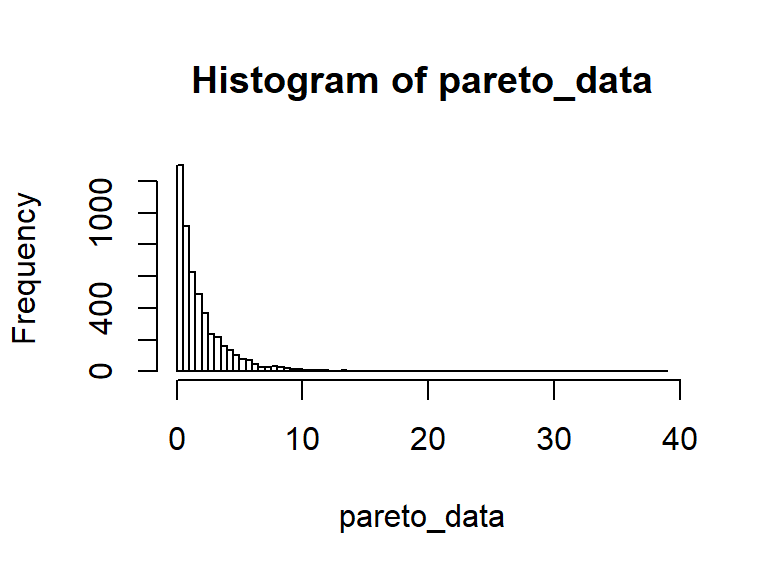min(pareto_data); max(pareto_data); d=max(pareto_data)-min(pareto_data);d
#>  0.0002192696
#>  38.56871
#>  38.56849
fit <- fitdist(pareto_data, "pareto", start = list(shape = 1, scale = 500))
fit
#> Fitting of the distribution ' pareto ' by maximum likelihood
#> Parameters:
#>       estimate Std. Error
#> shape 5.026907  0.4337688
#> scale 8.191676  0.8358576
res <- strata.data(pareto_data, h = 2, n=500) # a 2-strata solution
#> The program is running, it'll take some time!
summary(res)
#> _____________________________________________
#> Optimum Strata Boundaries for h = 2
#> Data Range: [0.0002192696, 38.56871] with d = 38.56849
#> Best-fit Frequency Distribution:  pareto
#> Parameter estimate(s):
#>    shape    scale
#> 5.016949 8.174580
#> ____________________________________________________
#>  Strata   OSB   Wh    Vh  WhSh  nh   Nh   fh
#>       1  3.03 0.79  0.64 0.632 232 3938 0.06
#>       2 38.57 0.21 11.87 0.732 268 1062 0.25
#>   Total       1.00 12.51 1.363 500 5000 0.10
#> ____________________________________________________

Similarly, in order to find the OSB and other quantities, we can apply the strata.distr() function to a Pareto Type II population. Let us assume from past knowledge that the study variable in the population follows Pareto Type II distribution with the given attributes such as the $$shape=5.05, scale=8.20, initial\,value=0.15$$ and $$distance=38.55$$. Then, if a sample of size $$n=500$$ is drawn from the population of size $$N=5000$$, we can execute the following command to obtain the results:

res <- strata.distr(h=2, initval=0.15, dist=38.55, distr = "pareto",
params = c(shape=5.05, scale=8.20), n=500, N=5000)
#> The program is running, it'll take some time!
summary(res)
#> _____________________________________________
#> Optimum Strata Boundaries for h = 2
#> Data Range: [0.15, 38.7] with d = 38.55
#> Best-fit Frequency Distribution:  pareto
#> Parameter estimate(s):
#> shape scale
#>  5.05  8.20
#> ____________________________________________________
#>  Strata  OSB   Wh    Vh  WhSh  nh   Nh   fh
#>       1 3.21 0.81  0.67 0.592 241 4057 0.06
#>       2 38.7 0.19 11.42 0.637 259  943 0.27
#>   Total      1.00 12.09 1.229 500 5000 0.10
#> ____________________________________________________

## 7.2 Stratification for a Survey Variable with Triangular Distribution

Let the study variable follow Triangular distribution on the domain of [$$a, b$$], its three-parameter probability density function with a state space $$y\geq 0$$ is given by: $\begin{equation} f(y) = \begin{cases} \dfrac{2(y-a)}{(b-a)(c-a)}; & y \in [a, c]\\[10pt] \dfrac{2(b-y)}{(b-a)(b-c)}; & y \in (c,b] \\ \end{cases} \tag{19} \end{equation}$

where $$a$$ is the location parameter, $$b$$ is the scale parameter and $$c$$ is the shape parameter of the distribution.

Then, formulating the problem as an MPP and solving the recurrence relations as discussed above for Pareto II variate, the OSB are obtained.

### 7.2.1 DP Solution for Triangular Distribution

To solve the MPP formulated for Triangular distribution (19), we apply the algorithm using the DP technique discussed in Section 4. The recurrence relations used to determine the OSB are given by:

For the first stage, $$k=1$$, at $$l_{1}^{*}=d_{1}$$:

$\begin{eqnarray} \Phi_{1}d_{1}&=& \textrm{Sqrt}\Biggl\{ \sum_{h=1}^{\lambda_{1}} \dfrac{d_{1}^{2}\sqrt{d_{1}^{2}+6(y_{0}-a)d_{1}+6(y_{0}-a)^{2}}}{3\sqrt{2}(b-a)(c-a)} + \sum_{h=\lambda_{2}}^{L}\dfrac{d_{1}^{2}\sqrt{6(b-y_{0})^{2}-6(b-y_{0})d_{1}+d_{1}^{2}}}{3\sqrt{2}(b-a)(b-c)}\Biggr\} \tag{20} \end{eqnarray}$

And for the stages $$k\geq2$$:

$\begin{eqnarray} \Phi_{k}d_{k}&=&\min_{0\leq l_{k}\leq d_{k}}\Biggl\{ \textrm{Sqrt}\Biggl\{\sum_{h=1}^{\lambda_{1}} \dfrac{l_{k}^{2}\sqrt{l_{k}^{2}+6a_{k}l_{k}+6a_{k}^{2}}}{3\sqrt{2}(b-a)(c-a)} +\sum_{h=\lambda_{2}}^{L}\dfrac{l_{k}^{2}\sqrt{6b_{k}^{2}-6b_{k}l_{k}+l_{k}^{2}}}{3\sqrt{2}(b-a)(b-c)}\Biggr\} + \Phi_{k-1}(d_{k}-l_{k})\Biggr\} \tag{21} \end{eqnarray}$

where $$a_{k}=d_{k}-l_{k}+y_{0}-a$$ and $$b_{k}=b-d_{k}+l_{k}-y_{0}+a$$.

Substituting the values of $$a$$, $$b$$, $$c$$, $$y_{0}$$ and $$d$$, the OSW ($$l_{h}^{*}$$) and the OSB ($$y_{h}^{*}=y_{h-1}^{*}-l_{h}^{*}$$) are obtained by executing the strata.data() function.

### 7.2.2 A Numerical Example for Triangular Distribution

Data on Mathematics marks of first year students in a University in Fiji, with a size of $$N=354$$ called ‘math’ data is used to demonstrate the application of the stratifyR package on a Triangular population. In this example, the variable final_marks’ is used - it exhibits 3-parameter Triangular distribution with the parameters: $$min = 6.205204$$, $$max=98.47165$$ and $$mode=53.999996$$. The minimum and maximum values in the ‘math’ data are $$[y_{0}, y_{L}] = [7, 97]$$, which implies that $$d=90$$.

To construct the OSB ($$h = 2$$) for the final_marks’ data with a fixed total sample size of $$150$$, we use the following code:

data(math)
final_marks <- math$final_marks hist(final_marks)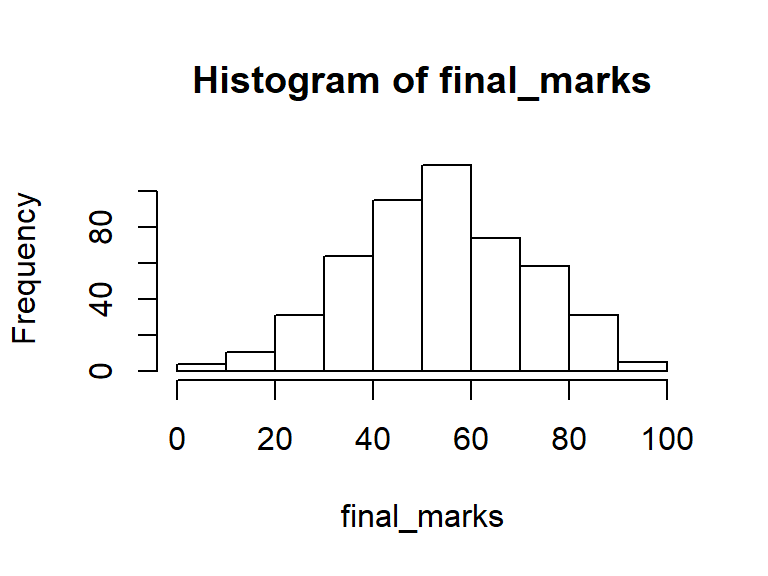res <- strata.data(final_marks, h = 2, n=150) # a 2-strata solution #> The program is running, it'll take some time! summary(res) #> _____________________________________________ #> Optimum Strata Boundaries for h = 2 #> Data Range: [7, 97] with d = 90 #> Best-fit Frequency Distribution: triangle #> Parameter estimate(s): #> min max mode #> 6.205204 98.471650 53.999996 #> ____________________________________________________ #> Strata OSB Wh Vh WhSh nh Nh fh #> 1 53.16 0.49 114.72 5.278 74 240 0.31 #> 2 97 0.51 116.01 5.463 76 247 0.31 #> Total 1.00 230.73 10.741 150 487 0.31 #> ____________________________________________________ Similarly, in order to find the OSB and other quantities, we can apply the strata.distr() function to a Triangular population. Based on the assumption from past knowledge that the population follows Triangular distribution with the given attributes such as the initial value, distance, parameters, etc., we can execute the following command to obtain the results: data(math) final_marks <- math$final_marks
eps = 1e-8; a <- min(final_marks); b <- max(final_marks); c=b-a# and with mode=54
a;b;c
#>  7
#>  97
#>  90
#find out the estimated parameters
fit <- fitdist(final_marks, distr = "triang", method="mle", lower=c(0,0),
start = list(min = a-eps, max = b+eps, mode = 54))
fit
#> Fitting of the distribution ' triang ' by maximum likelihood
#> Parameters:
#>       estimate Std. Error
#> min   6.205364         NA
#> max  98.469797         NA
#> mode 53.999994         NA
# 2-strata solution
res <- strata.distr(h=2, initval=7, dist=90, distr = "triangle",
params = c(min=6.205364, max=98.469797, mode=53.999994), n=150, N=352)
#> The program is running, it'll take some time!
summary(res)
#> _____________________________________________
#> Optimum Strata Boundaries for h = 2
#> Data Range: [7, 97] with d = 90
#> Best-fit Frequency Distribution:  triangle
#> Parameter estimate(s):
#>       min       max      mode
#>  6.205364 98.469797 53.999994
#> ____________________________________________________
#>  Strata   OSB  Wh     Vh   WhSh  nh  Nh   fh
#>       1 53.16 0.5 122.23  5.525  76 176 0.43
#>       2    97 0.5 113.22  5.316  74 176 0.42
#>   Total       1.0 235.45 10.841 150 352 0.43
#> ____________________________________________________

## 7.3 Stratification for a Survey Variable with Right-Triangular Distribution

If a study variable follows the Right-Triangular distribution on the domain of [$$a, b$$], its two-parameter probability density function is given by:

$\begin{equation} f(y) = \begin{cases} \dfrac{2(b-y)}{(b-a)^2}; & y \in [a, b]\\ 0; & otherwise \\ \end{cases} \tag{22} \end{equation}$

where $$a$$ is the location parameter and $$b$$ is the scale parameter of the distribution.

Note that the Right-Triangular distribution is a special case of the Triangular distribution discussed in Section 7.2 where the parameters $$a=c$$, i.e., minimium value is equal to the mode. Thus, the density function (22) is a two-parameter distribution.

### 7.3.1 DP Solution for Right-Triangular Distribution

To solve the MPP formulated for Right-Triangular distribution (22), we apply the algorithm using the DP technique discussed in Section 4. The recurrence relations used to determine the OSB are given by:

For the first stage, $$k=1$$, at $$l_{1}^{*}=d_{1}$$:

$\begin{eqnarray} \Phi_{1}d_{1}&=& \textrm{Sqrt}\Biggl\{ \left[\dfrac{d_{1}(2(y_{0}-a)-d_{1})}{(b-a)^{2}}\right]^{2}\times\left[\dfrac{d_{1}^{2}(d_{1}^{2}-6(y_{0}-a)d_{1}+6a_{h}^{2})}{18(2(y_{0}-a)-d_{1})^{2}}\right]\Biggr\} \tag{23} \end{eqnarray}$

And for the stages $$k\geq2$$:

$\begin{eqnarray} \Phi_{k}d_{k}&=&\min_{0\leq l_{k}\leq d_{k}}\Biggl\{ \textrm{Sqrt}\Biggl\{\left[\dfrac{l_{k}(2a_{k}-l_k)}{(b-a)^{2}}\right]^{2} \times\left[\dfrac{l_{k}^{2}(l_{k}^{2}-6a_{k}l_{k}+6a_{k}^{2})}{18(2a_{k}-l_{k})^{2}}\right]\Biggr\} + \Phi_{k-1}(d_{k}-l_{k})\Biggr\} \tag{24} \end{eqnarray}$

where $$a_{k}=b-d_{k}+l_{k}-y_{0}$$.

Substituting the values of $$a$$, $$b$$, $$y_{0}$$ and $$d$$, the OSW ($$l_{h}^{*}$$) and the OSB ($$y_{h}^{*}=y_{h-1}^{*}-l_{h}^{*}$$) are obtained by executing the strata.data() function.

### 7.3.2 A Numerical Example for Right-Triangular Distribution

A data following Right-Triangular distribution, of size $$N=5000$$, was simulated to demonstrate the application of the stratifyR package. The simulated data takes in three parameters where $$a=c$$ to indicate that it’s a Right-Triangular distribution. Upon fitting the data, its best-fit distribution exhibits a three-parameter Triangular distribution with the parameters $$min = 1.987776$$, $$max=7.935599$$ and $$mode=2.026685$$. Note that because the $$min$$ and $$mode$$ parameters are very close to each and not exactly the same, it is treated as a Triangular distribution even thought the data simulated was for a Right-Triangular distribution. The minimum and maximum values in the simulated data are $$[y_{0}, y_{L}] = [2.000052, 7.83871]$$ with $$d=5.838658$$.

To construct the OSB ($$h = 2$$) for the Right-Triangular data with a fixed total sample size of $$500$$, we use the following code:

#Generate RT data
set.seed(12546)
data <- rtriangle(n=1000, a=2, b=8, c=2) #right-triangular since a=c
hist(data)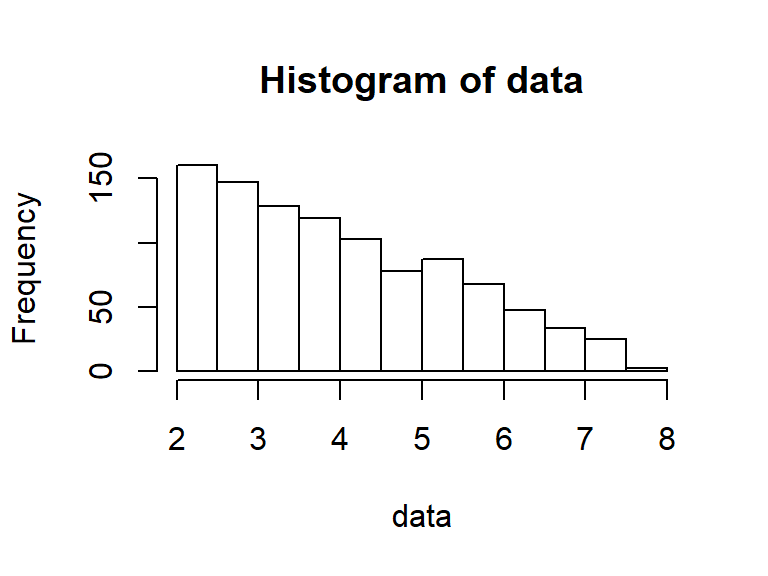res <- strata.data(data, h = 2, n=500) # a 2-strata solution
#> The program is running, it'll take some time!
summary(res)
#> _____________________________________________
#> Optimum Strata Boundaries for h = 2
#> Data Range: [2.000052, 7.83871] with d = 5.838658
#> Best-fit Frequency Distribution:  triangle
#> Parameter estimate(s):
#>      min      max     mode
#> 1.987776 7.935599 2.026685
#> ____________________________________________________
#>  Strata  OSB   Wh   Vh  WhSh  nh   Nh   fh
#>       1  4.1 0.57 0.36 0.339 233  568 0.41
#>       2 7.84 0.43 0.81 0.388 267  432 0.62
#>   Total      1.00 1.16 0.727 500 1000 0.50
#> ____________________________________________________

We see in the above example that the simulated data is a Right-Triangular distribution, however, when data is fitted using MLE method in the package, it turns out that it best-fits Triangular distribution because the $$min$$ is not exactly equal to $$mode$$.

Similarly, in order to find the OSB and other quantities, we can apply the strata.distr() function to a Right-Triangular population. Based on the assumption from past knowledge that the population follows Right-Triangular distribution with the given attributes such as the initial value, distance, parameters, etc., we can execute the following command to obtain the results:

res <- strata.distr(h=2, initval=1.007202, dist=0.992781, distr = "rtriangle",
params = c(min=2, max=10, mode=2), n=500, N=1000)
#> The program is running, it'll take some time!
summary(res)
#> _____________________________________________
#> Optimum Strata Boundaries for h = 2
#> Data Range: [1.007202, 1.999983] with d = 0.992781
#> Best-fit Frequency Distribution:  rtriangle
#> Parameter estimate(s):
#>  min  max mode
#>    2   10    2
#> ____________________________________________________
#>  Strata OSB    Wh   Vh  WhSh   nh   Nh   fh
#>       1 1.5 -0.13 0.02 0.019 -130 -130 1.00
#>       2   2  1.13 0.02 0.019  630 1130 0.56
#>   Total      1.00 0.04 0.038  500 1000 0.50
#> ____________________________________________________

The results show that this fits a two-paramter Right-Triangular distribution. Do note that in the above command, one has to specify all three parameters $$min=2, max=10$$ and $$mode=2$$, even for a Right-Triangular distribution, where $$min=mode$$.

## 7.4 Stratification for a Survey Variable with Weibull Distribution

If the study variable follows the Weibull distribution on the interval $$[y_{0}, y_{L}]$$, its two-parameter probability density function with a state space $$y\geq 0$$ is given by: $\begin{equation} f(y; \theta, r)=\dfrac{r}{\theta}\left(\dfrac{y}{\theta}\right)^{r-1}e^{-\left(y/\theta\right)^{r}}, \;\;\;\;\;y\geq 0 \tag{25} \end{equation}$

where $$r > 0$$ is the shape parameter and $$\theta > 0$$ is the scale parameter of the distribution.

### 7.4.1 DP Solution for Weibull Distribution

To solve the MPP formulated for Weibull distribution (25), we apply the algorithm using the DP technique discussed in Section 4. The recurrence relations used to determine the OSB are given by:

For the first stage, $$k=1$$, at $$l_{1}^{*}=d_{1}$$:

$\begin{eqnarray} \Phi_{1}d_{1}&=& \textrm{Sqrt}\Biggl\{ \theta^{2}\,\Gamma\left(1+\dfrac{2}{r} \right)\left[e^{-\left(\frac{y_{0}}{\theta}\right)^{r}}-e^{-\left(\frac{d_{1}+y_{0}}{\theta}\right)^{r}}\right] \nonumber \\[10pt] &&\times \left[Q\left(1+\dfrac{2}{r},\left(\dfrac{y_{0}}{\theta}\right)^{r}\right)- Q\left(1+\dfrac{2}{r},\left(\dfrac{d_{1}+y_{0}}{\theta}\right)^{r}\right)\right]\nonumber\\[10pt] &&-\theta^{2}\left[\Gamma\left(1+\dfrac{1}{r}\right)\left [Q\left(1+\dfrac{1}{r},\left(\dfrac{y_{0}}{\theta}\right)^{r}\right)\right.\right.\nonumber\\[10pt] &&-Q\left.\left.\left(1+\dfrac{1}{r},\left(\dfrac{d_{1}+y_{0}}{\theta}\right)^{r}\right)\right]\right]^{2}\Biggr\} \tag{26} \end{eqnarray}$

And for the stages $$k\geq2$$:

$\begin{eqnarray} \Phi_{k}d_{k}&=&\min_{0\leq l_{k}\leq d_{k}}\Biggl\{ \textrm{Sqrt}\Biggl\{ \theta^{2}\,\Gamma\left(1+\dfrac{2}{r} \right)\left[e^{-\left(\frac{d_{k}-l_{k}+y_{0}}{\theta}\right)^{r}}-e^{-\left(\frac{d_{k}+y_{0}}{\theta}\right)^{r}}\right]\nonumber\\[10pt] &&\times \left[Q\left(1+\dfrac{2}{r},\left(\dfrac{d_{k}-l_{k}+y_{0}}{\theta}\right)^{r}\right)- Q\left(1+\dfrac{2}{r},\left(\dfrac{d_{k}+y_{0}}{\theta}\right)^{r}\right)\right]\nonumber\\[15pt] &&-\theta^{2}\left[\Gamma\left(1+\dfrac{1}{r}\right)\left [Q\left(1+\dfrac{1}{r},\left(\dfrac{d_{k}-l_{k}+y_{0}}{\theta}\right)^{r}\right)\right.\right.\nonumber\\[15pt] &&-Q\left.\left.\left(1+\dfrac{1}{r},\left(\dfrac{d_{k}+y_{0}}{\theta}\right)^{r}\right)\right]\right]^{2}\Biggr\}+\Phi_{k-1}(d_{k}-l_{k})\Biggr\}. \tag{27} \end{eqnarray}$

The recurrence relations (26) and (27) are solved using the DP technique to determine the OSB.

### 7.4.2 A Numerical Example for Weibull Distribution

A health data of size $$N=724$$, called ‘anaemia’ data (Reddy, Khan, and Rao (2014)), is used to demonstrate the application of the stratifyR package on Weibull population. The ‘anaemia’ data comes from the National Nutritional Survey on the Micronutrient Status of Women in Fiji" and has many variables such as level of Iron, Folate, Zinc, etc. In this example, the variable Iron is used since it exhibits a 2-parameter Weibull distribution with the shape and scale parameters as $$r = 2.144586$$ and $$\theta = 13.790744$$ respectively. The minimum and maximum values are $$[y_{0}, y_{L}] = [1.5, 34.7]$$, which implies that $$d=33.2$$.

To construct the OSB ($$h = 2$$) for the Iron data with a fixed total sample size of $$500$$, we use the following codes:

data(anaemia) #using the anaemia data
Iron <- anaemia$Iron hist(Iron)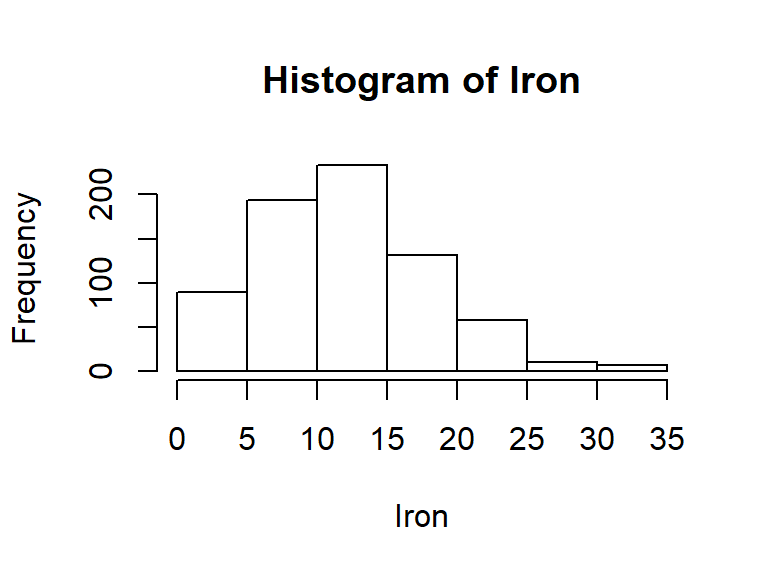res <- strata.data(Iron, h = 2, n=500) # a 2-strata solution #> The program is running, it'll take some time! summary(res) #> _____________________________________________ #> Optimum Strata Boundaries for h = 2 #> Data Range: [1.5, 34.7] with d = 33.2 #> Best-fit Frequency Distribution: weibull #> Parameter estimate(s): #> shape scale #> 2.144879 13.792245 #> ____________________________________________________ #> Strata OSB Wh Vh WhSh nh Nh fh #> 1 12.74 0.6 9.03 1.789 255 431 0.59 #> 2 34.7 0.4 18.11 1.722 245 293 0.84 #> Total 1.0 27.14 3.511 500 724 0.69 #> ____________________________________________________ Similarly, in order to find the OSB and other quantities, we can apply the strata.distr() function to a Weibull population. Based on the assumption from past knowledge that the population follows Weibull distribution with the given attributes such as the initial value, distance, parameters, etc., we can execute the following command to obtain the results: res <- strata.distr(h=2, initval=2.9, dist=55.9, distr = "weibull", params = c(shape=2.144586, scale=13.790744), n=500, N=5000) #> The program is running, it'll take some time! summary(res) #> _____________________________________________ #> Optimum Strata Boundaries for h = 2 #> Data Range: [2.9, 58.8] with d = 55.9 #> Best-fit Frequency Distribution: weibull #> Parameter estimate(s): #> shape scale #> 2.144586 13.790744 #> ____________________________________________________ #> Strata OSB Wh Vh WhSh nh Nh fh #> 1 13.05 0.59 7.49 1.516 239 2943 0.08 #> 2 58.8 0.41 16.28 1.660 261 2057 0.13 #> Total 1.00 23.77 3.176 500 5000 0.10 #> ____________________________________________________ ## 7.5 Stratification for a Survey Variable with Gamma Distribution If the study variable follows the Gamma distribution (i.e., $$y\sim \Gamma(r, \theta)$$) on the interval $$[y_{0}, y_{L}]$$, it has the following two-parameter probability density function: $\begin{equation} f(y;r,\theta)=\dfrac{1}{\theta^{r}\Gamma(r)}\,y^{r-1}e^{-\dfrac{y}{\theta}},\;\;\;\;\;\;\;y>0;\;\;r,\theta>0, \tag{28} \end{equation}$ where is a shape parameter and $$\theta$$ is the scale parameter and $$\Gamma(r)$$ is a Gamma function defined by $\begin{equation}\label{222} \Gamma(r)=\int_{0}^{\infty}t^{r-1}e^{-t}\,dt,\;\;\;\;\;\; r > 0. \tag{29} \end{equation}$ The function in equation $$(\ref{222})$$ is also defined by an upper incomplete gamma function $$\Gamma(r,x)$$ and a lower incomplete gamma function $$\gamma(r,x)$$, respectively, as follows: $\begin{eqnarray} \Gamma(r,y)=\int_{y}^{\infty}t^{r-1}e^{-t}\,dt;\label{223} \tag{30}\\ \gamma(r,y)=\int_{0}^{y}t^{r-1}e^{-t}\,dt.\label{224} \tag{31} \end{eqnarray}$ There also exist regularized/normalised incomplete Gamma functions which give a value restricted between $$0$$ and $$1$$ and can be stated as: $\begin{eqnarray} Q(r,y)=\dfrac{1}{\Gamma(r)}\int_{y}^{\infty}t^{r-1}e^{-t}\,dt,\;\;\;\;\;\;r,y>0;\;\;\Gamma(r)\neq0;\label{225} \tag{32}\\ P(r,y)=\dfrac{1}{\Gamma(r)}\int_{0}^{y}t^{r-1}e^{-t}\,dt,\;\;\;\;\;\;r,y>0;\;\;\Gamma(r)\neq0,\label{226} \tag{33} \end{eqnarray}$ where $$Q(r,y)$$ denotes the Upper Regularized Incomplete Gamma function while $$P(r,y)$$ denotes regularized Lower Incomplete Gamma function (Abramowitz and Stegun (1972), Chapter 6). Note that $$Q(r,y)=1-P(r,y)$$. ### 7.5.1 DP Solution for Gamma Distribution To solve the MPP formulated for Gamma distribution (28), we apply the algorithm using the DP technique discussed in Section 4. The recurrence relations used to determine the OSB are given by: For the first stage, $$k=1$$, at $$l_{1}^{*}=d_{1}$$: $\begin{eqnarray} \Phi_{1}d_{1}&=& \textrm{Sqrt}\Biggl\{ \theta^{2}\,r(r+1)\left[ Q\left(r,\dfrac{y_{0}}{\theta}\right)-Q\left(r,\dfrac{d_{1}+y_{0}}{\theta}\right)\right]\nonumber\\[10pt] &&\times\left[Q\left(r+2,\dfrac{y_{0}}{\theta}\right)-Q\left(r+2,\dfrac{d_{1}+y_{0}}{\theta}\right) \right]\nonumber\\[10pt] &&-\theta^{2}r^{2}\left[ Q\left(r+1,\dfrac{y_{0}}{\theta}\right)-Q\left(r+1,\dfrac{d_{1}+y_{0}}{\theta}\right)\right]^{2}\Biggr\} \tag{34} \end{eqnarray}$ And for the stages $$k\geq2$$: $\begin{eqnarray} \Phi_{k}d_{k}&=&\min_{0\leq l_{k}\leq d_{k}}\Biggl\{ \textrm{Sqrt}\Biggl\{ \theta^{2}\,r(r+1)\left[ Q\left(r,\dfrac{d_{k}-l_{k}+y_{0}}{\theta}\right.\right)\nonumber\\[10pt] &&-\left.Q\left(r,\dfrac{d_{k}+y_{0}}{\theta}\right)\right] \times\left[ Q\left(r+2,\dfrac{d_{k}-l_{k}+y_{0}}{\theta}\right.\right)\nonumber\\[10pt] &&-\left.Q\left(r+2,\dfrac{d_{k}+y_{0}}{\theta}\right) \right]-\theta^{2}r^{2}\times\left[ Q\left(r+1,\dfrac{d_{k}-l_{k}+y_{0}}{\theta}\right)\right.\nonumber\\[10pt] &&-\left.Q\left(r+1,\dfrac{d_{k}+y_{0}}{\theta}\right)\right]^{2}\Biggr\}+\Phi_{k-1}(d_{k}-l_{k})\Biggr\}. \tag{35} \end{eqnarray}$ The recurrence relations (34) and (35) are solved using the DP technique to determine the OSB. ### 7.5.2 A Numerical Example for Gamma Distribution Again, the health data of size $$N=724$$, derived from the ‘National Nutritional Survey’ on the ‘Micronutrient Status of Women in Fiji’ is used to demonstrate the application of the stratifyR package on Gamma population. In this example, the variable Folate is used since it exhibits a 2-parameter Gamma distribution with the shape and scale parameters as $$r = 6.9922$$ and $$\theta = 2.5785$$ respectively. The minimum and maximum values are $$[y_{0}, y_{L}] = [4.9, 45.4]$$, which implies that $$d=40.5$$. To construct the OSB ($$h = 2$$) for the Folate data with a fixed total sample size of $$500$$, we use the following codes: data(anaemia) Folate <- anaemia$Folate
hist(Folate)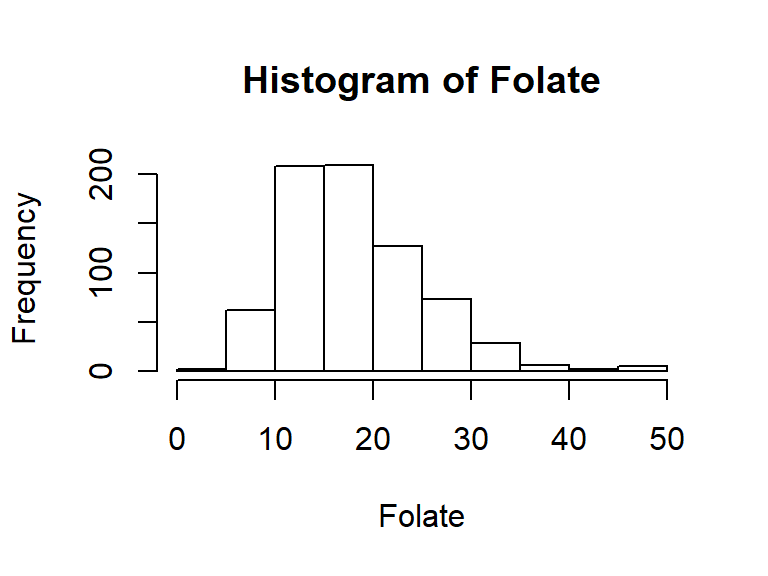res <- strata.data(Folate, h = 2, n=500) # a 2-strata solution
#> The program is running, it'll take some time!
summary(res)
#> _____________________________________________
#> Optimum Strata Boundaries for h = 2
#> Data Range: [4.9, 45.4] with d = 40.5
#> Best-fit Frequency Distribution:  gamma
#> Parameter estimate(s):
#>     shape      rate
#> 6.9922028 0.3878246
#> ____________________________________________________
#>  Strata   OSB   Wh    Vh  WhSh  nh  Nh   fh
#>       1 18.83 0.61 10.78 2.005 244 442 0.55
#>       2  45.4 0.39 29.05 2.099 256 282 0.91
#>   Total       1.00 39.83 4.104 500 724 0.69
#> ____________________________________________________

Similarly, in order to find the OSB and other quantities, we can apply the strata.distr() function to a Gamma population. Based on the assumption from past knowledge that the population follows Gamma distribution with the given attributes such as the initial value, distance, parameters, etc., we can execute the following command to obtain the results:

res <- strata.distr(h=2, initval=0.5, dist=50, distr = "gamma",
params = c(shape=3.835768, rate=0.340328), n=500, N=12000)
#> The program is running, it'll take some time!
summary(res)
#> _____________________________________________
#> Optimum Strata Boundaries for h = 2
#> Data Range: [0.5, 50.5] with d = 50
#> Best-fit Frequency Distribution:  gamma
#> Parameter estimate(s):
#>    shape     rate
#> 3.835768 0.340328
#> ____________________________________________________
#>  Strata   OSB   Wh    Vh  WhSh  nh    Nh   fh
#>       1 12.18 0.63  6.95 1.653 247  7522 0.03
#>       2  50.5 0.37 20.50 1.689 253  4478 0.06
#>   Total       1.00 27.45 3.342 500 12000 0.04
#> ____________________________________________________

## 7.6 Stratification for a Survey Variable with Exponential Distribution

If the study variable follows the Exponential distribution on the interval $$[y_{0}, y_{L}]$$, its one-parameter probability density function is given by:

$\begin{equation} f(y; \lambda)= \begin{cases} \lambda\mathrm{e}^{-\lambda y}; & y > 0\\ \;\;\;\;0; & elsewhere \\ \end{cases} \tag{36} \end{equation}$

where $$\lambda$$ is the continuous rate parameter (or the inverse scale parameter).

### 7.6.1 DP Solution for Exponential Distribution

To solve the MPP formulated for Exponential distribution (36), we apply the algorithm using the DP technique discussed in Section 4. The recurrence relations used to determine the OSB are given by:

For the first stage, $$k=1$$, at $$l_{1}^{*}=d_{1}$$:

$\begin{eqnarray} \Phi_{1}d_{1}&=& \textrm{Sqrt}\Biggl\{ \left(e^{-\lambda y_{0}}\right)^{2}\left[\dfrac{1}{\lambda^2}\left(1-e^{-\lambda l_{k}}\right)^{2}-l_{k}^{2}e^{-\lambda l_{k}}\right]\Biggr\} \tag{37} \end{eqnarray}$

And for the stages $$k\geq2$$:

$\begin{eqnarray} \Phi_{k}d_{k}&=&\min_{0\leq l_{k}\leq d_{k}}\Biggl\{ \textrm{Sqrt}\Biggl\{ \left(e^{-\lambda (d_{k}-l_{k}+y_{0})}\right)^{2}\left[\dfrac{1}{\lambda^2}\left(1-e^{-\lambda l_{k}}\right)^{2}-l_{k}^{2}e^{-\lambda l_{k}}\right]\Biggr\} + \Phi_{k-1}(d_{k}-l_{k})\Biggr\} \tag{38} \end{eqnarray}$

Substituting the values of $$\lambda$$, $$y_{0}$$ and $$d$$, the OSW ($$l_{h}^{*}$$) and the OSB ($$y_{h}^{*}=y_{h-1}^{*}-l_{h}^{*}$$) are obtained by executing the strata.data() function.

### 7.6.2 A Numerical Example for Exponential Distribution

A data following Exponential distribution (herein called $$Exp$$ data), of size $$N=10000$$ was simulated to demonstrate the application of the stratifyR package on an Exponential population. The data exhibits an Exponential distribution with the parameter $$rate = 1.359205$$. The minimum and maximum values in the simulated data are $$[y_{0}, y_{L}] = [5.747904e-05, 8.016871]$$, which implies that $$d=8.016814$$.

To construct the OSB ($$h = 2$$) for the data that follows Exponential distribution with a fixed total sample size of $$500$$, we use the following codes:

set.seed(28951)
data <- rexp(5000, rate = 1.36)
hist(data)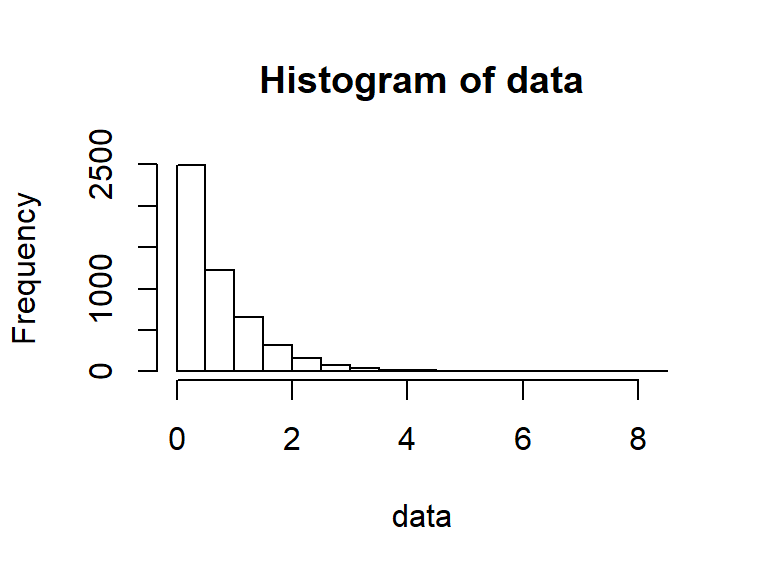res <- strata.data(data, h = 2, n=500) # a 2-strata solution
#> The program is running, it'll take some time!
summary(res)
#> _____________________________________________
#> Optimum Strata Boundaries for h = 2
#> Data Range: [5.747904e-05, 8.016871] with d = 8.016814
#> Best-fit Frequency Distribution:  exp
#> Parameter estimate(s):
#>     rate
#> 1.359205
#> ____________________________________________________
#>  Strata  OSB   Wh   Vh  WhSh  nh   Nh   fh
#>       1 0.93 0.72 0.07 0.187 235 3596 0.07
#>       2 8.02 0.28 0.57 0.212 265 1404 0.19
#>   Total      1.00 0.64 0.399 500 5000 0.10
#> ____________________________________________________

Similarly, in order to find the OSB and other quantities, we can apply the strata.distr() function to a Exponential population. Based on the assumption from past knowledge that the population follows Exponential distribution with the given attributes such as the initial value, distance, parameters, etc., we can execute the following command to obtain the results:

set.seed(28951)
data <- rexp(5000, rate = 1.36)
min(data); max(data); d=max(data)-min(data);d
#>  5.747904e-05
#>  8.016871
#>  8.016814
fit <- fitdist(data, distr="exp", method="mle")
fit
#> Fitting of the distribution ' exp ' by maximum likelihood
#> Parameters:
#>      estimate Std. Error
#> rate 1.359205 0.01922205
res <- strata.distr(h=2, initval=5.748e-05, dist=8.017, distr = "exp",
params = c(rate=1.36), n=500, N=5000) #a 2-strata solution
#> The program is running, it'll take some time!
summary(res)
#> _____________________________________________
#> Optimum Strata Boundaries for h = 2
#> Data Range: [5.748e-05, 8.017057] with d = 8.017
#> Best-fit Frequency Distribution:  exp
#> Parameter estimate(s):
#> rate
#> 1.36
#> ____________________________________________________
#>  Strata  OSB   Wh   Vh  WhSh  nh   Nh   fh
#>       1 0.93 0.72 0.07 0.185 235 3584 0.07
#>       2 8.02 0.28 0.54 0.208 265 1416 0.19
#>   Total      1.00 0.60 0.392 500 5000 0.10
#> ____________________________________________________

## 7.7 Stratification for a Survey Variable with Uniform Distribution

If the study variable follows the Uniform distribution on the interval $$[y_{0}, y_{L}]$$, its two-parameter probability density function is given by:

$\begin{equation} f(y; a,b)= \begin{cases} \dfrac{1}{b-a}; & y > 0\\ \;\;\;\;0; & otherwise \\ \end{cases} \tag{39} \end{equation}$

where $$a$$ and $$b$$ are the continuous boundary parameters, i.e., $$a$$ and $$b$$ are the minimum and maximum values respectively.

### 7.7.1 DP Solution for Uniform Distribution

To solve the MPP formulated for Uniform distribution (39), we apply the algorithm using the DP technique discussed in Section 4. The recurrence relations used to determine the OSB are given by:

For the first stage, $$k=1$$, at $$l_{1}^{*}=d_{1}$$:

$\begin{eqnarray} \Phi_{1}d_{1}&=& \textrm{Sqrt}\Biggl\{ \sum\limits_{h=1}^{L} \dfrac{d_{1}^{2}}{2\sqrt{3}(b-a)}\Biggr\} \tag{40} \end{eqnarray}$

And for the stages $$k\geq2$$:

$\begin{eqnarray} \Phi_{k}d_{k}&=&\min_{0\leq l_{k}\leq d_{k}}\Biggl\{ \textrm{Sqrt}\Biggl\{ \sum\limits_{h=1}^{L} \dfrac{l_{k}^{2}}{2\sqrt{3}(b-a)}\Biggr\}+ \Phi_{k-1}(d_{k}-l_{k})\Biggr\} \tag{41} \end{eqnarray}$

Substituting the values of $$a$$, $$b$$, $$y_{0}$$ and $$d$$, the OSW ($$l_{h}^{*}$$) and the OSB ($$y_{h}^{*}=y_{h-1}^{*}-l_{h}^{*}$$) are obtained by executing the strata.data() function.

### 7.7.2 A Numerical Example for Uniform Distribution

A data following Uniform distribution of size $$N=5000$$ was simulated to demonstrate the application of the stratifyR package on a Uniform population. The data for Uniform distribution is simulated with the parameters $$min = 2$$ and $$max=15$$. When fitted, the minimum and maximum values in the simulated data are $$[y_{0}, y_{L}] = [2.006522, 14.99764]$$, which implies that $$d=12.99112$$.

To construct the OSB ($$h = 2$$) for the Uniformly-distributed data with a fixed total sample size of $$450$$, we use the following codes:

set.seed(15669)
data <- runif(5000, min = 2, max = 15)
hist(data)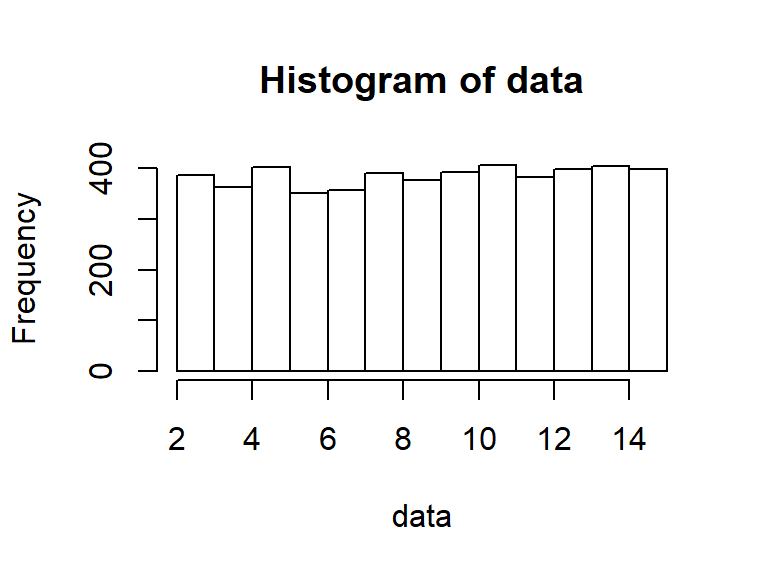res <- strata.data(data, h = 2, n=450) # a 2-strata solution
#> The program is running, it'll take some time!
summary(res)
#> _____________________________________________
#> Optimum Strata Boundaries for h = 2
#> Data Range: [2.006522, 14.99764] with d = 12.99112
#> Best-fit Frequency Distribution:  triangle
#> Parameter estimate(s):
#>          min          max         mode
#> 2.275043e-06 1.540557e+01 1.340821e+01
#> ____________________________________________________
#>  Strata  OSB   Wh   Vh  WhSh  nh   Nh   fh
#>       1 9.87 0.59 5.21 1.354 312 2966 0.11
#>       2   15 0.41 2.19 0.601 138 2034 0.07
#>   Total      1.00 7.40 1.956 450 5000 0.09
#> ____________________________________________________

Note that the above results indicate that the best-fit distribution is Triangular and not Uniform. This is because when stratifyR package assesses the data to ascertain the best-fit distribution, it is not able to calculate its AIC value for Uniform distribution. Hence, the distribution that gives the lowest AIC is taken to be the best-fit distribution. Since AIC for Uniform distribution is not available, the next best-fit distribution (Triangular) is be chosen. The results, you would find, are still quite accurate!

Similarly, we can apply the strata.distr() function for the Uniform distribution where the arguments can be assumed from past knowledge. To construct the OSB for $$h = 2$$ for a hypothetical variable that follows Uniform distribution (with parameters $$min=3$$ and $$max=15$$) with a fixed total sample size of $$450$$ from a population of $$5000$$, the following command is used:

# For a hypothetical uniform distribution, it does give a result
res <- strata.distr(h=2, initval=3, dist=12, distr = "unif",
params = c(min=3, max=15), n=450, N=5000)
#> The program is running, it'll take some time!
summary(res)
#> _____________________________________________
#> Optimum Strata Boundaries for h = 2
#> Data Range: [3, 15] with d = 12
#> Best-fit Frequency Distribution:  unif
#> Parameter estimate(s):
#> min max
#>   3  15
#> ____________________________________________________
#>  Strata OSB Wh Vh  WhSh  nh   Nh   fh
#>       1   9  0  3 0.866 225 2500 0.09
#>       2  15  0  3 0.866 225 2500 0.09
#>   Total      1  6 1.732 450 5000 0.09
#> ____________________________________________________

## 7.8 Stratification for a Survey Variable with Normal Distribution

If the study variable follows the Normal distribution on the interval $$[y_{0}, y_{L}]$$, it has the following two-parameter probability density function: $\begin{equation} f(y;\mu, \sigma)=\dfrac{1}{\sigma\sqrt{2\pi}}\,exp\left\{-\dfrac{1}{2}\left(\dfrac{y-\mu}{\sigma}\right)^{2}\right\},\;\;\;\;\;\ -\infty < y < \infty \tag{42} \end{equation}$

where $$\sigma > 0$$ is a scale parameter and $$\mu$$ is the location parameter.

The following definitions of error function are worth noting since they are needed to simplify the integrations used to derive the stratum weight, mean and variance due to normal distribution. $\begin{eqnarray} erf(z) = \dfrac{2}{\sqrt{\pi}}\int_{0}^{z}exp\left\{-y^{2}\right\}\,dy \tag{43} \end{eqnarray}$ It can also be written as $\begin{eqnarray}\label{erf} \dfrac{1}{\sqrt{2\pi}}\int_{0}^{z}exp\left\{-\dfrac{1}{2}y^{2}\right\}\,dy = \dfrac{1}{2}\,erf\left(\dfrac{z}{\sqrt{2}}\right) \tag{44} \end{eqnarray}$

### 7.8.1 DP Solution for Normal Distribution

To solve the MPP formulated for Normal distribution (42), we apply the algorithm using the DP technique discussed in Section 4. The recurrence relations used to determine the OSB are given by:

For the first stage, $$k=1$$, at $$l_{1}^{*}=d_{1}$$:

$\begin{eqnarray} \Phi_{1}d_{1}&=& \textrm{Sqrt}\Biggl\{ \dfrac{\sigma^{2}}{2\sqrt{2\pi}} \left[erf\left(\dfrac{d_{1}+y_{0}-\mu}{\sigma\sqrt{2}}\right) - erf\left(\dfrac{y_{0}-\mu}{\sigma\sqrt{2}}\right)\right]\nonumber \\[10pt] && \times\left[\left(\dfrac{y_{0}-\mu}{\sigma}\right)exp\left(-\left(\dfrac{y_{0}-\mu}{\sigma\sqrt{2}}\right)^{2}\right)- \left(\dfrac{d_{1}+y_{0}-\mu}{\sigma}\right)exp\left(-\left(\dfrac{d_{1}+y_{0}-\mu}{\sigma\sqrt{2}}\right)^{2}\right)\right]\nonumber\\[10pt] && + \dfrac{\sigma^{2}}{4}\left[erf\left(\dfrac{d_{1}+y_{0}-\mu}{\sigma\sqrt{2}}\right) - erf\left(\dfrac{y_{0}-\mu}{\sigma\sqrt{2}}\right)\right]^{2}\nonumber\\[10pt] && - \dfrac{\sigma^{2}}{2\pi}\left[exp\left(-\left(\dfrac{y_{0}-\mu}{\sigma\sqrt{2}}\right)^{2}\right)- exp\left(-\left(\dfrac{d_{1}+y_{0}-\mu}{\sigma\sqrt{2}}\right)^{2}\right)\right]^{2}\Biggr\} \tag{45} \end{eqnarray}$

And for the stages $$k\geq2$$:

$\begin{eqnarray} \Phi_{k}d_{k}&=&\min_{0\leq l_{k}\leq d_{k}}\Biggl\{ \textrm{Sqrt}\Biggl\{ \dfrac{\sigma^{2}}{2\sqrt{2\pi}} \left[ erf\left(\dfrac{d_{k}+y_{0}-\mu}{\sigma\sqrt{2}}\right) - erf\left(\dfrac{(d_{k}-l_{k}+y_{0})-\mu}{\sigma\sqrt{2}}\right) \right]\nonumber \\[10pt] && \times\left[\left(\dfrac{(d_{k}-l_{k}+y_{0})-\mu}{\sigma}\right)exp\left(-\left(\dfrac{(d_{k}-l_{k}+y_{0})-\mu}{\sigma\sqrt{2}}\right)^{2}\right)\right. \nonumber\\[10pt] && - \left. \left(\dfrac{d_{k}+y_{0}-\mu}{\sigma}\right)exp\left(-\left(\dfrac{d_{k}+y_{0}-\mu}{\sigma\sqrt{2}}\right)^{2}\right)\right]\nonumber\\[10pt] && + \dfrac{\sigma^{2}}{4}\left[erf\left(\dfrac{d_{k}+y_{0}-\mu}{\sigma\sqrt{2}}\right) - erf\left(\dfrac{(d_{k}-l_{k}+y_{0})-\mu}{\sigma\sqrt{2}}\right)\right]^{2}\nonumber\\[10pt] && - \dfrac{\sigma^{2}}{2\pi}\left[exp\left(-\left(\dfrac{(d_{k}-l_{k}+y_{0})-\mu}{\sigma\sqrt{2}}\right)^{2}\right)-exp\left(-\left(\dfrac{d_{k}+y_{0}-\mu}{\sigma\sqrt{2}}\right)^{2}\right)\right]^{2}\Biggr\} + \Phi_{k-1}(d_{k}-l_{k})\Biggr\} \tag{46} \end{eqnarray}$

Substituting the values of $$\mu$$, $$\sigma$$, $$y_{0}$$ and $$d$$, the OSW ($$l_{h}^{*}$$) and the OSB ($$y_{h}^{*}=y_{h-1}^{*}-l_{h}^{*}$$) are obtained by executing the strata.data() function.

### 7.8.2 A Numerical Example for Normal Distribution

A data following Normal distribution (herein called $$Norm$$ data), of size $$N=5000$$ was simulated to demonstrate the application of the stratifyR package on a Normal population. The data exhibits an Normal distribution with the parameters $$mean = 16.010776$$ and $$sd=1.662357$$. The minimum and maximum values in the simulated data are $$[y_{0}, y_{L}] = [9.923816, 22.51267]$$, which implies that $$d=10.62118$$.

To construct the OSB for $$h = 2$$ using the $$Norm$$ data with a fixed total sample size of $$580$$, the command below can be used:

set.seed(89821)
data <- rnorm(5000, mean = 16, sd = 1.65)
hist(data)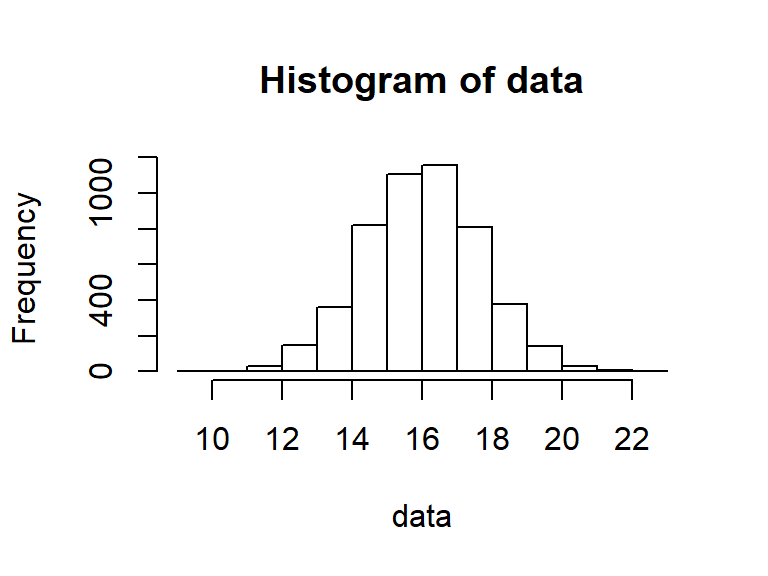res <- strata.data(data, h = 2, n=500) #construct a 2-strata solution
#> The program is running, it'll take some time!
summary(res)
#> _____________________________________________
#> Optimum Strata Boundaries for h = 2
#> Data Range: [9.923816, 22.51267] with d = 12.58885
#> Best-fit Frequency Distribution:  norm
#> Parameter estimate(s):
#>      mean        sd
#> 16.010776  1.662357
#> ____________________________________________________
#>  Strata   OSB  Wh   Vh  WhSh  nh   Nh  fh
#>       1 16.01 0.5 0.98 0.493 244 2489 0.1
#>       2 22.51 0.5 1.05 0.515 256 2511 0.1
#>   Total       1.0 2.03 1.009 500 5000 0.1
#> ____________________________________________________

Similarly, in order to find the OSB and other quantities, we can apply the strata.distr() function to a Normal population. Based on the assumption from past knowledge that the population follows Normal distribution with the given attributes such as the initial value, distance, parameters, etc., we can execute the following command to obtain the results:

set.seed(89821)
data <- rnorm(5000, mean = 16, sd = 1.65)
min(data); max(data); d=max(data)-min(data);d
#>  9.923816
#>  22.51267
#>  12.58885
fit <- fitdist(data, distr="norm", method="mle")
fit
#> Fitting of the distribution ' norm ' by maximum likelihood
#> Parameters:
#>       estimate Std. Error
#> mean 16.010776 0.02350928
#> sd    1.662357 0.01662355
res <- strata.distr(h=2, initval=9.923816, dist=12.58885, distr = "norm",
params = c(mean=16.010776, sd=1.662357), n=500, N=5000)
#> The program is running, it'll take some time!
summary(res)
#> _____________________________________________
#> Optimum Strata Boundaries for h = 2
#> Data Range: [9.923816, 22.51267] with d = 12.58885
#> Best-fit Frequency Distribution:  norm
#> Parameter estimate(s):
#>      mean        sd
#> 16.010776  1.662357
#> ____________________________________________________
#>  Strata   OSB  Wh Vh WhSh  nh   Nh  fh
#>       1 16.01 0.5  1  0.5 250 2501 0.1
#>       2 22.51 0.5  1  0.5 250 2499 0.1
#>   Total       1.0  2  1.0 500 5000 0.1
#> ____________________________________________________

## 7.9 Stratification for a Survey Variable with Log-Normal Distribution

If the study variable follows the Log-normal distribution on the interval $$[y_{0}, y_{L}]$$, it has the following two-parameter probability density function:

$\begin{equation} f(y;\mu,\sigma)=\dfrac{1}{y\sigma\sqrt{2\pi}}\,exp\left\{-\dfrac{1}{2}\left(\dfrac{ln(y)-\mu}{\sigma}\right)^{2}\right\},\;\;\;\;\;\ y>0 \tag{47} \end{equation}$

where $$\sigma > 0$$ is a scale parameter and $$\mu$$ is the location parameter.

### 7.9.1 DP Solution for Log-Normal Distribution

To solve the MPP formulated for Log-Normal distribution (47), we apply the algorithm using the DP technique discussed in Section 4. The recurrence relations used to determine the OSB are given by:

For the first stage, $$k=1$$, at $$l_{1}^{*}=d_{1}$$:

$\begin{eqnarray} \Phi_{1}d_{1}&=& \textrm{Sqrt}\Biggl\{\frac{1}{4}exp\left(2\mu+2\sigma^{2}\right)\left[ erf\left(\frac{ln(d_{1}+y_{0})-\mu-2\sigma^{2}}{\sigma\sqrt{2}}\right)\right.\nonumber \\[10pt] && \left. - erf\left(\frac{ln(y_{0})-\mu-2\sigma^{2}}{\sigma\sqrt{2}}\right) \right] \left[ erf\left(\frac{ln(d_{1}+y_{0})-\mu}{\sigma\sqrt{2}}\right) - erf\left(\frac{ln(y_{0})-\mu}{\sigma\sqrt{2}}\right)\right]\nonumber \\[10pt] && -\frac{1}{4}exp\left(2\mu+\sigma^{2}\right)\left[erf\left(\frac{ln(d_{1}+y_{0})-\mu-\sigma^{2}}{\sigma\sqrt{2}}\right) - erf\left(\frac{ln(y_{0})-\mu-\sigma^{2}}{\sigma\sqrt{2}}\right) \right]^{2}\Biggr\} \tag{48} \end{eqnarray}$

And for the stages $$k\geq2$$:

\[\begin{eqnarray} \Phi_{k}d_{k}&=&\min_{0\leq l_{k}\leq d_{k}}\Biggl\{ \textrm{Sqrt}\Biggl\{ \frac{1}{4}exp\left(2\mu+2\sigma^{2}\right)\left[erf\left(\frac{ln(d_{k}+y_{0})-\mu-2\sigma^{2}}{\sigma\sqrt{2}}\right)\right.\nonumber \\[10pt] && - \left. erf\left(\frac{ln(d_{k}-l_{k}+y_{0})-\mu-2\sigma^{2}}{\sigma\sqrt{2}}\right) \right] \left[ erf\left(\frac{ln(d_{k}+y_{0})-\mu}{\sigma\sqrt{2}}\right)\right.\nonumber \\[10pt] && -\left. erf\left(\frac{ln(d_{k}-l_{k}+y_{0})-\mu}{\sigma\sqrt{2}}\right) \right]-\frac{1}{4}exp\left(2\mu+\sigma^{2}\right)\nonumber \\[10pt] &&\times \left[ erf\left(\frac{ln(d_{k}+y_{0})-\mu-\sigma^{2}}{\sigma\sqrt{2}}\right) - erf\left(\frac{ln(d_{k}-l_{